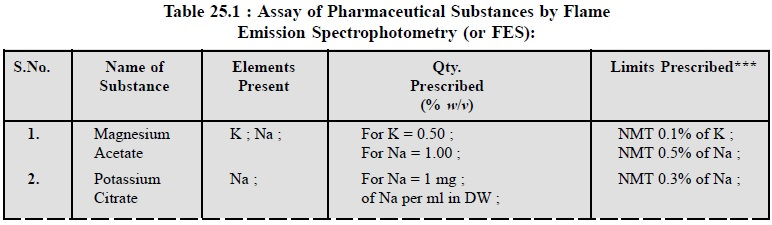Home | | Pharmaceutical Drug Analysis | Applications of Flame Emission Spectroscopy in Pharmaceutical Analysis

# Applications of Flame Emission Spectroscopy in Pharmaceutical Analysis

A few typical example of ‘flame-emission spectroscopy’ are given below.

APPLICATIONS OF FLAME EMISSION SPECTROSCOPY IN PHARMACEUTICAL ANALYSIS

A few typical example of ‘flame-emission spectroscopy’ are given below.

## 1. ASSAY OF SODIUM, POTASSIUM AND CALCIUM IN BLOOD SERUM AND WATER

(i) Standard potassium and sodium solutions, approximately 500 ppm : Weigh accurately 0.95 g of dried KCl and 1.25 g of dried NaCl into separate 1-litre volumetric flasks. Dissolve in water and dilute to the mark.

(ii) Standard calcium solution, approximately 500 ppm : Weigh accurately 1.25 g of CaCO3, which has been dried at 110°C, into a 500-ml breaker. Add about 200 ml of DW and 10 ml of conc. HCl. Cover the breaker with a watch-glass during addition of acid to prevent loss of solution as CO2 is evolved. After the solution is complete, transfer it quantitatively into a 1-litre volumetric flask and dilute to the mark with DW.

(iii) Radiation buffer* for sodium determination : Prepare a saturated solution with reagent-grade CaCl2, KCl, MgCl2, in that order.

(iv) Radiation buffer for potassium determination : Prepare a saturated solution with reagent-grade NaCl, CaCl2 and MgCl2, in that order.

(v) Radiation buffer for calcium determination : Prepare a saturated solution with reagent-grade NaCl, KCl, MgCl2 in that order.

Procedure

(a) Preparation of working curves : Transfer 5 ml of the appropriate radiation buffer to each series of 100-ml volumetric flasks. Add a volume of the standard solution which will cover a concentra-tion ranging between 0 to 100 ppm. Dilute to 100 ml with DW and mix well. Measure the emis-sion intensity of these samples by taking at least three readings for each. Between each set of measurements, aspirate DW through the burner. Correct the average values for background lumi-nosity, and prepare a working curve from these data.

(b) Analysis of blood serum/water sample : Prepare aliquot portions of the sample as described in the above paragraph (a). If necessary, use a standard to calibrate the response of the spectrometer to the working curve. Then measure the emission intensity for the unknown. After correcting the data for background, determine the concentration by comparison with the working curve.

## 2. ASSAY OF BARIUM, POTASSIUM AND SODIUM IN CALCIUM ACETATE

The technique of flame emission spectroscopy is used to determine the concentration of Ba, K, and Na ions by measuring the intensity of emission at a specific wavelength by the atomic vapour of the element generated from calcium acetate i.e., by introducing its solution into a flame.

### 2.1. For Emission Measurements

Introduce water into the atomic vapour generator, adjust the instrument reading to zero, introduce the most concentrated solution into the generator and adjust the sensitivity to give a suitable reading ; again introduce water or the prescribed solution into the generator and when the reading is constant readjust, if necessary, to zero.

### 2.2. Method of Standard Addition

The various steps are as follows :

Place in each of not fewer than three similar graduated flasks equal volumes of the solutions of the substance being examined, prepared as follows :

(a) Prepare a 5.0% w/v solution and use barium solution ASp*, suitably diluted with water to prepare the standard solution.

(b) Prepare a 1.25% w/v solution and use potassium solution ASp**, suitably diluted with mater, to prepare the standard solution.

(c) Prepare a 1.0% w/v solution and use sodium solution ASp***, suitably diluted with water, to prepare the standard solution.

Add to all but one these flasks a measured quantity of the specified standard solution (marked *; **; ***; above) to produce a series of solution containing increasing amounts of the element being determined.

Dilute the contents of each flask to the required volume with water.

After having calibrated the instrument as directed above, introduce each solution into the genera-tor three times and record the steady reading. If the generator is a flame, wash the apparatus thoroughly with water ; if a furnace is used fire it after each introduction.

Plot the mean of the readings against concentration on a graph the axes of which intersect at zero added element and zero reading.

Extrapolate the straight line joining the points until it meets the extrapolated concentration axis. The distance between this point and the intersection of the axes represents the concentration of the element (e.g., Mg, K, Na) being determined in the solution of the substance being examined.

### 2.3. Limits of Elements present in Calcium Acetate Sample

Mg  : Not more than 500 ppm of Mg ;

K : Not more than 0.1% of K, and

Na : Not more than 0.5% of Na.

## 3. COGNATE ASSAYS

The following substance, namely : magnesium acetate ; potassium citrate ; potassium hydroxide ; potassium nitrate and sodium chloride can also be assayed for their respective elements as shown in Table 25.1 below. However, all the respective solutions of the said pharmaceutical substance and their standard solutions must be prepared as prescribed in BP (1993) strictly to obtain the best results.Study Material, Lecturing Notes, Assignment, Reference, Wiki description explanation, brief detail
Pharmaceutical Drug Analysis: Flame Spectroscopy : Applications of Flame Emission Spectroscopy in Pharmaceutical Analysis |